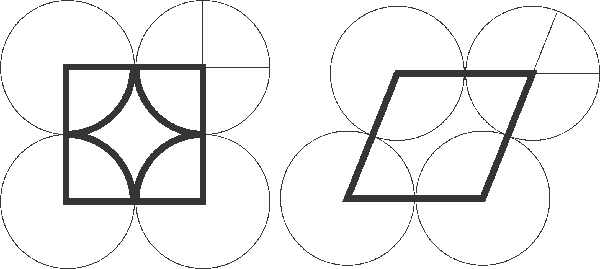SEARCH HOMEMath Central Quandaries & QueriesQuestion from varsha, a student: four circular cardboard pieces each of radius 7cm are placed in such a way that each piece touches two other pieces.find the area enclosed by the four pieces.Hi Varsha, This question has not got an answer! If the discs are placed at the corners of a square, you can see that the area between them is the
area of the square (what is that?) minus the area of four quarter circles (and what is that?)

However, the discs can be put at the corners of a rhombus, which has a smaller area (anywhere between 1 and $\large \frac{sqr{3}}{2} \normalsize \approx 0.87$ times that of the square, depending on its shape). As you can see, the missing sectors still add to exactly one disc.Good Hunting!
RDMath Central is supported by the University of Regina and The Pacific Institute for the Mathematical Sciences.